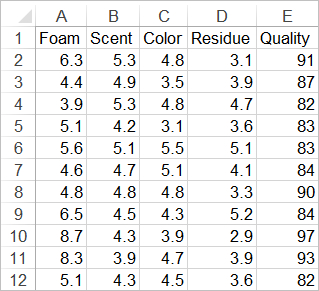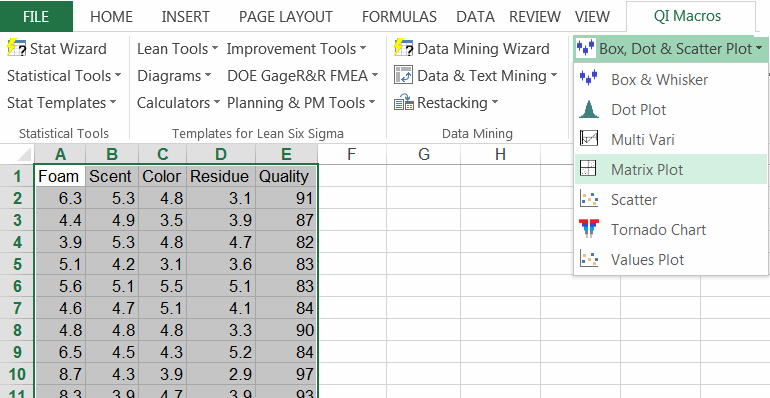# Want a Scatter Plot Matrix or Matrix Plot in Excel?

## QI Macros Makes it Easy to Create a Matrix Plot in Excel## Use a Matrix Plot to evaluate the cause-effect when you have two or more variables

A scatter plot matrix is used to evaluate the correlation or cause-effect relationship (if any) between two or more variables. The matrix plot is really just a series of mini scatter diagrams.

In this example we want to evaluate the cause-effect relationship between several factors (foam, scent, color, and residue) on the perceived quality of shampoo.To create a scatter plot matrix in Excel using QI Macros just select your data, and then Matrix Plot from QI Macros menu:Column E represents the cause-effect relationship between each individual factor and its effect (or not) on the perceived quality. In this, example there is no correlation between any of the factors and the perceived quality.

### Summary

When you're investigating cause-effect links between two or more indicators, the scatter plot matrix can quickly identify correlations. If the points are tightly clustered along a line like machine gun stitching, then there's probably a strong correlation. If it looks more like a shotgun blast, there is no correlation. If you aren't sure, run a scatter diagram for the two variables and look at the R2 value. If R 2 is greater than .80, there is a strong correlation.